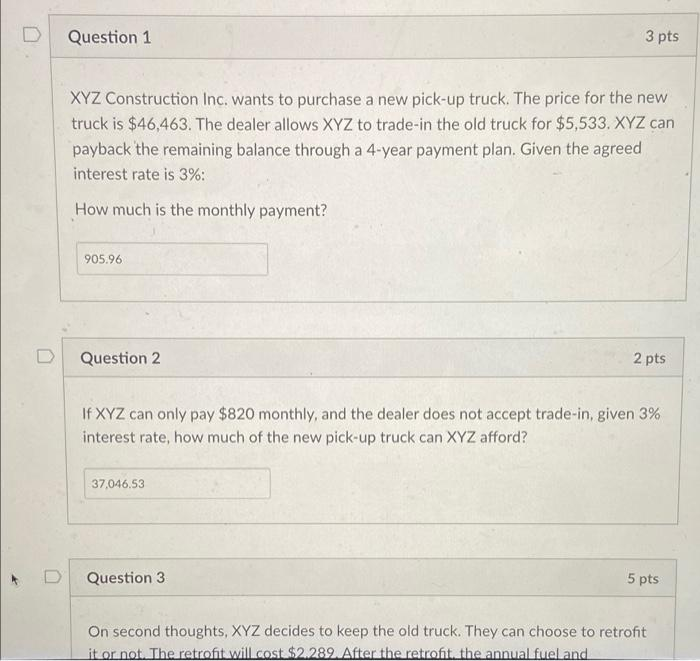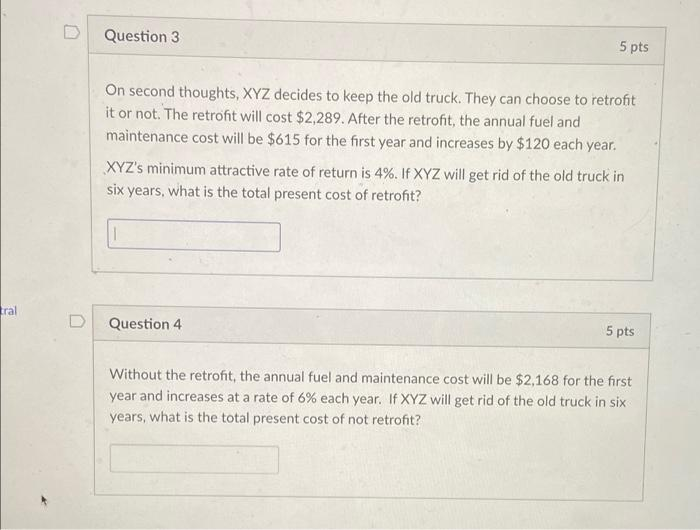Home / Expert Answers / Finance / mathrm-xyz-construction-inc-wants-to-purchase-a-new-pick-up-truck-the-price-for-the-new-t-pa276

# (Solved): $$\mathrm{XYZ}$$ Construction Inc. wants to purchase a new pick-up truck. The price for the new t ...$$\mathrm{XYZ}$$ Construction Inc. wants to purchase a new pick-up truck. The price for the new truck is $$\ 46,463$$. The dealer allows $$X Y Z$$ to trade-in the old truck for $$\ 5,533$$. $$X Y Z$$ can payback the remaining balance through a 4 -year payment plan. Given the agreed interest rate is $$3 \%$$ : How much is the monthly payment? Question 2 2 pts If $$\mathrm{XYZ}$$ can only pay $$\ 820$$ monthly, and the dealer does not accept trade-in, given $$3 \%$$ interest rate, how much of the new pick-up truck $$\operatorname{can} X Y Z$$ afford? Question 3 5 pts On second thoughts, XYZ decides to keep the old truck. They can choose to retrofit it or not. The retrofit will cost $$\ 2.289$$. After the retrofit, the annual fuel and On second thoughts, $$X Y Z$$ decides to keep the old truck. They can choose to retrofit it or not. The retrofit will cost $$\ 2,289$$. After the retrofit, the annual fuel and maintenance cost will be $$\ 615$$ for the first year and increases by $$\ 120$$ each year. $$X Y Z$$ 's minimum attractive rate of return is $$4 \%$$. If $$X Y Z$$ will get rid of the old truck in six years, what is the total present cost of retrofit? Question 4 5 pts Without the retrofit, the annual fuel and maintenance cost will be $$\ 2,168$$ for the first year and increases at a rate of $$6 \%$$ each year. If $$X Y Z$$ will get rid of the old truck in six years, what is the total present cost of not retrofit?

We have an Answer from Expert